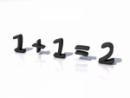### Numerical Ability Exam – Syllabus & Topics to Prepare

Be it school or college, mathematics & numbers have been the most feared subjects for many.The trend continues in bank exams too as many assume numerical ability to be tough.So, in continuation of our series about preparing for common written exam, we shall look at the syllabus for numerical ability this week.Numerical ability is all about calculation of numbers.Unlike quantitative aptitude, this paper would require simple mind calculations only.Given the job profile of a bank employee, test of numerical ability mainly aims to find out how fast you arrive at the correct answer.

The clerk exam pattern depicts this section to have 50 questions each carrying 1 mark.(50 x 1 = 50 marks) & each wrong answer shall reduce 0.25 marks.

Topics to cover: Based on questions repeatedly asked in previous banking exams, we have provided a predictive list of important chapters for preparing.

1. Numerical problems (missing no.,series completion etc)
2. Percentages (like profit & loss %, marks,shares etc.,)
3. Market price,Cash price & Expenditure problems.
4. Time & Work, Speed & Distance problems
5. Fraction, Ratios, Average & Volume
6. Factoring (LCM, HCF..)
7. Mensuration formulas (asking you to calculate length, breadth or height of square,rectangle cube etc)
8. Simple interest & Compound interest (find missing variable or direct question)

Preparations:

• Know about BODMAS (Brackets, Order, Divide, Multiply, Add, Subtract) & workout problems implementing the rule.
• Use common sense to solve questions that are real life oriented (like distance, age, time)
• Calculators are not permitted inside the exam hall.Hence learn shortcut methods or formulas to solve a question quickly.
• Time management is essential while attending mathematical problems.So tryout some mock tests with the same number of questions while preparing.
• Practice as many model papers & previous year question papers with a clock - because time management is essential for mathematical problems.

Candidates who are weak in maths shall buy books like pratiyogita darpan, kiran publications or other workbooks/guides & practice min.5 yrs solved  question papers to memorize the concept.

Getting ready for IBPS Clerk CWE? Checkout the Topics for general & banking awareness exam

Get FREE Email Alerts on Bank Jobs/Exams & other Govt Recruitment news, Tips to Prepare & Books ->